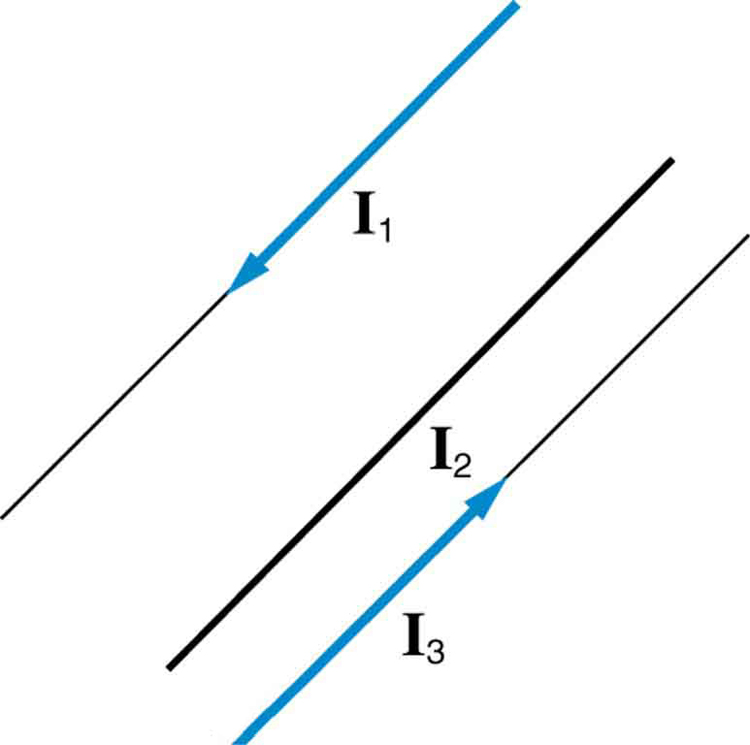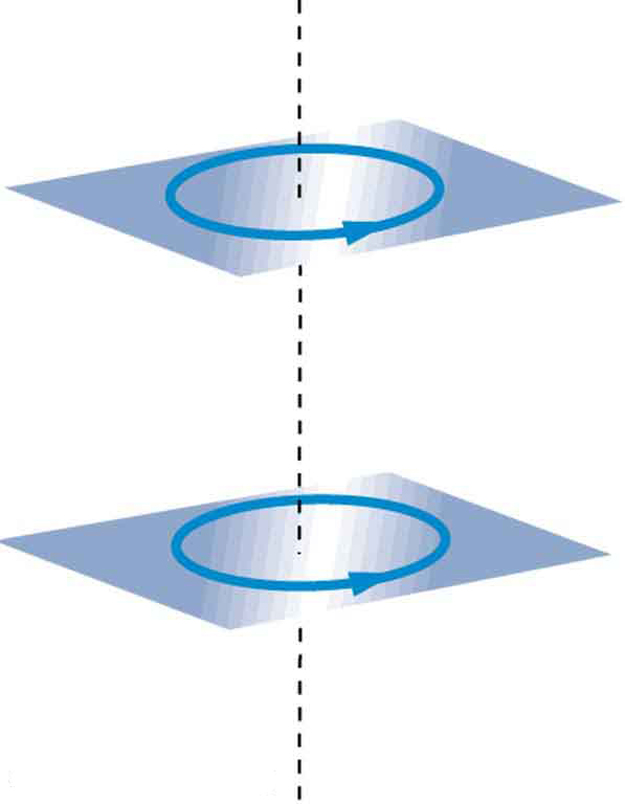# 22.10 Magnetic force between two parallel conductors  (Page 2/2)

 Page 2 / 2

Since ${\mu }_{0}$ is exactly $4\pi ×{\text{10}}^{-7}\phantom{\rule{0.25em}{0ex}}T\cdot \text{m/A}$ by definition, and because $\text{1 T}=\text{1 N/}\left(A\cdot m\right)$ , the force per meter is exactly $2×{\text{10}}^{-7}\phantom{\rule{0.25em}{0ex}}\text{N/m}$ . This is the basis of the operational definition of the ampere.

## The ampere

The official definition of the ampere is:

One ampere of current through each of two parallel conductors of infinite length, separated by one meter in empty space free of other magnetic fields, causes a force of exactly $2×{\text{10}}^{-7}\phantom{\rule{0.25em}{0ex}}\text{N/m}$ on each conductor.

Infinite-length straight wires are impractical and so, in practice, a current balance is constructed with coils of wire separated by a few centimeters. Force is measured to determine current. This also provides us with a method for measuring the coulomb. We measure the charge that flows for a current of one ampere in one second. That is, $1 C=1 A\cdot s$ . For both the ampere and the coulomb, the method of measuring force between conductors is the most accurate in practice.

## Section summary

• The force between two parallel currents ${I}_{1}$ and ${I}_{2}$ , separated by a distance $r$ , has a magnitude per unit length given by
$\frac{F}{l}=\frac{{\mu }_{0}{I}_{1}{I}_{2}}{2\mathrm{\pi r}}.$
• The force is attractive if the currents are in the same direction, repulsive if they are in opposite directions.

## Conceptual questions

Is the force attractive or repulsive between the hot and neutral lines hung from power poles? Why?

If you have three parallel wires in the same plane, as in [link] , with currents in the outer two running in opposite directions, is it possible for the middle wire to be repelled by both? Attracted by both? Explain.Three parallel coplanar wires with currents in the outer two in opposite directions.

Suppose two long straight wires run perpendicular to one another without touching. Does one exert a net force on the other? If so, what is its direction? Does one exert a net torque on the other? If so, what is its direction? Justify your responses by using the right hand rules.

Use the right hand rules to show that the force between the two loops in [link] is attractive if the currents are in the same direction and repulsive if they are in opposite directions. Is this consistent with like poles of the loops repelling and unlike poles of the loops attracting? Draw sketches to justify your answers.Two loops of wire carrying currents can exert forces and torques on one another.

If one of the loops in [link] is tilted slightly relative to the other and their currents are in the same direction, what are the directions of the torques they exert on each other? Does this imply that the poles of the bar magnet-like fields they create will line up with each other if the loops are allowed to rotate?

Electric field lines can be shielded by the Faraday cage effect. Can we have magnetic shielding? Can we have gravitational shielding?

## Problems&Exercises

(a) The hot and neutral wires supplying DC power to a light-rail commuter train carry 800 A and are separated by 75.0 cm. What is the magnitude and direction of the force between 50.0 m of these wires? (b) Discuss the practical consequences of this force, if any.

(a) 8.53 N, repulsive

(b) This forceis repulsiveand thereforethere isnever arisk thatthe twowires willtouch andshort circuit.

The force per meter between the two wires of a jumper cable being used to start a stalled car is 0.225 N/m. (a) What is the current in the wires, given they are separated by 2.00 cm? (b) Is the force attractive or repulsive?

A 2.50-m segment of wire supplying current to the motor of a submerged submarine carries 1000 A and feels a 4.00-N repulsive force from a parallel wire 5.00 cm away. What is the direction and magnitude of the current in the other wire?

400 A in the opposite direction

The wire carrying 400 A to the motor of a commuter train feels an attractive force of $4\text{.}\text{00}×{\text{10}}^{-3}\phantom{\rule{0.25em}{0ex}}\text{N/m}$ due to a parallel wire carrying 5.00 A to a headlight. (a) How far apart are the wires? (b) Are the currents in the same direction?

An AC appliance cord has its hot and neutral wires separated by 3.00 mm and carries a 5.00-A current. (a) What is the average force per meter between the wires in the cord? (b) What is the maximum force per meter between the wires? (c) Are the forces attractive or repulsive? (d) Do appliance cords need any special design features to compensate for these forces?

(a) $1\text{.}\text{67}×{\text{10}}^{-3}\phantom{\rule{0.25em}{0ex}}\text{N/m}$

(b) $3\text{.}\text{33}×{\text{10}}^{-3}\phantom{\rule{0.25em}{0ex}}\text{N/m}$

(c) Repulsive

(d) No, these are very small forces

[link] shows a long straight wire near a rectangular current loop. What is the direction and magnitude of the total force on the loop?

Find the direction and magnitude of the force that each wire experiences in [link] (a) by, using vector addition.

(a) Top wire: $2\text{.}\text{65}×{\text{10}}^{-4}\phantom{\rule{0.25em}{0ex}}\text{N/m}$ s, $\text{10}\text{.}9º$ to left of up

(b) Lower left wire: $3\text{.}\text{61}×{\text{10}}^{-4}\phantom{\rule{0.25em}{0ex}}\text{N/m}$ , $\text{13}\text{.}9º$ down from right

(c) Lower right wire: $3\text{.}\text{46}×{\text{10}}^{-4}\phantom{\rule{0.25em}{0ex}}\text{N/m}$ , $\text{30}\text{.}0º$ down from left

Find the direction and magnitude of the force that each wire experiences in [link] (b), using vector addition.

A stone is dropped down a well, if it take 5 seconds to reach the water, how dip is the well
an aircraft at as steady velocity of 70m/so eastwards at a height of 800me drops a package of supplies .a, how long will it take for the package to rich the ground? b, how fast will it be going as it lands?
what is hypothesis theory law
Tamba
physics is the science of measurement
What is physics
what is physics
Obaro
Good question! Physics is the study of the nature world . Does this help?
Yonn
physics is the study of matter in relations to energy.
Enoch
physics is the science of measurements
Jide
physics is a science concern with nature and properties of matter and energy
Ugomma
what is a parallelogram law of motion?
Nancy
Definition for physics
It deal with matter and relation to energy
Soughie
physics is the Study of matter in relation to energy.
albert
physics is a natural science that study matter its behaviour and relation to energy.
mohammed
physic tells us more about quantities and measurement also
Kelly
life as we know it that can be measured and calculated
Jesus
what is a reference frame
what is anatomy in relation to physics
how does half life exist
The amount of time it takes a radioactive isotope to decay into a stable isotope is different for each radioactive isotope, and is characterized by its “half-life”. An isotope's half-life is the amount of time it takes for half the number of atoms of that isotope to decay to another isotope.
Nardine
what is the difference between Mass and weight
Pjustin
mass is constant while weight varies. unit of mass is kg, unit of weight is newton
Faith
how can a coin float in water and what principle governs it
in my opinion that work of surface tension but restrictions on coin is that coin do not break surface energy of molecules but some days before scientists prove that's another types of force
Aman
which force hold floating coins together thats my confusion
Aman
how can a coin float in water and what principle governs it
why many of the coin floating in water
what is hook's law
provided the elastic limit is not exceeded,the extension produce in an elastic material is equal to the applied force
Mahmud
What is Andromeda
👡
Mahmud
What are the international agreed system of unit for physical measurements
m, kg, s
Faith
kg , m, s
Faith
si
Aman
meter , kilogram , second
Kelly
international system of units is agreed system because more units are not in mks system so si exist with ampere unit
Aman
what is thermodynasmics
what is electromagnetic force
Femkid
Electromagnetic force means a force that deals with electric and magnetic
NebilByByBy